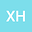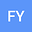loading page

Existence of Periodic Solutions for a Class of Fourth-order Difference Equation
••Xiaoling Han
Northwest Normal University
Author Profile## Abstract

We apply the continuation theorem of Mawhin to ensure that a fourth-order nonlinear difference equation of the form $$\Delta^4 u(k-2) -a(k)u^{\alpha}(k)+b(k)u^{\beta}(k)=0,$$ with periodic boundary conditions possesses at least one nontrivial positive solution, where $\Delta u(k)=u(k+1)-u(k)$ is the forward difference operator, $\alpha,\beta\in\mathbb{N}^+$ and $\alpha\neq\beta$. $a(k),b(k)$ are $T$-periodic functions and $a(k)b(k)>0$. As applications, we will give some examples to illustrate the application of these theorems.